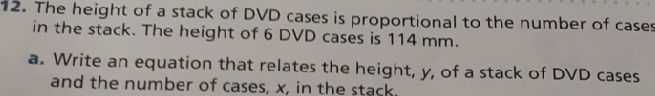### ¿Todavía tienes preguntas de matemáticas?

Pregunte a nuestros tutores expertos
Algebra
Pregunta12. The height of a stack of DVD cases is proportional to the number of cases in the stack. The height of $$6$$ DVD cases is $$114 mm$$ .

a. Write an equation that relates the heignt, $$y ,$$ of a stack of DVD cases and the number of cases, $$x$$ , in the stack.